Share

# Construct an Isosceles Triangle in Which the Lengths of Each of Its Equal Sides is 6.5 Cm and the Angle Between Them is 110°. - CBSE Class 7 - Mathematics

ConceptConstructing a Triangle When the Lengths of Two Sides and the Measure of the Angle Between Them Are Known. (Sas Criterion)

#### Question

Construct an isosceles triangle in which the lengths of each of its equal sides is 6.5 cm and the angle between them is 110°.

#### Solution

An isosceles triangle PQR has to be constructed with PQ = QR = 6.5 cm. A rough sketch of the required triangle can be drawn as follows.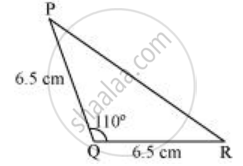The steps of construction are as follows.

1) Draw the line segment QR of length 6.5 cm.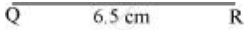2) At point Q, draw a ray QX making an angle 110° with QR.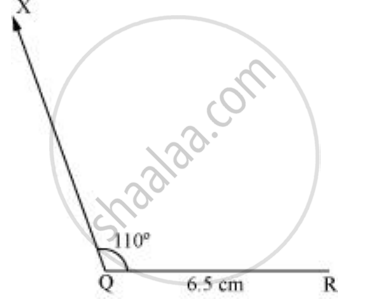3) Taking Q as centre, draw an arc of 6.5 cm radius. It intersects QX at point P.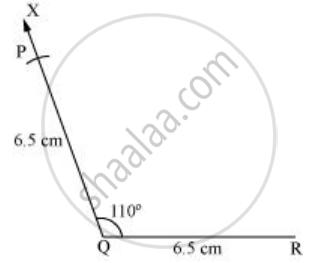4) Join P to R to obtain the required triangle PQR.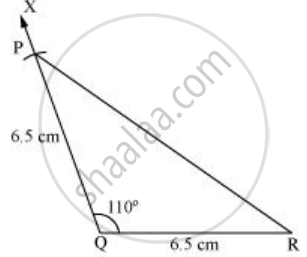Is there an error in this question or solution?

#### APPEARS IN

NCERT Solution for Mathematics for Class 7 (2018 to Current)
Chapter 10: Practical Geometry
Ex. 10.30 | Q: 2 | Page no. 200
Solution Construct an Isosceles Triangle in Which the Lengths of Each of Its Equal Sides is 6.5 Cm and the Angle Between Them is 110°. Concept: Constructing a Triangle When the Lengths of Two Sides and the Measure of the Angle Between Them Are Known. (Sas Criterion).
S